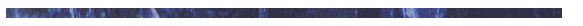# APPENDIX 3, DETERMINING UPPER SURFACE CAMBER OF A WING.Relevant considerations for this computation are:

1. Chord or chord line - an imaginary straight line joining the extremities of the leading edge and the trailing edge. For the purpose of this computation, this imaginary line will be measured midway between the tip of the wing and the wing root.

2. Camber - the curvature of a wing. For the purpose of this computation, only the camber of the upper surface (above the chord line) of the wing is considered.

STEP ONE - Determine the mid-point of the wing and measure the mean chord line.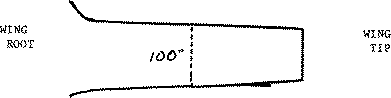STEP TWO - At the mid-point of the wing, determine the chord line horizontal position on the airfoil profile (assuming straight line from the extreme forward point on the leading edge to the extreme rearward point of the trailing edge).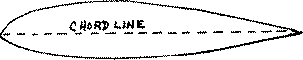STEP THREE - Measure the distance from the chord line to the highest point of camber on the upper surface.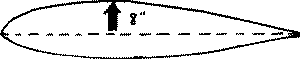STEP FOUR - Divide the measurement obtained in step one into the measurement obtained in step three.
```             Example: Upper surface camber    =   8 inches
Mean chord line length = l00 Inches = 8% camber
```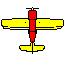Back to the Ultralight home page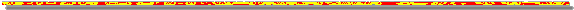Jon N. Steiger / stei0302@cs.fredonia.edu / SUNY College at Fredonia